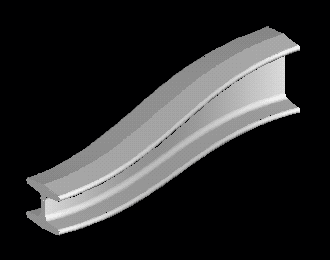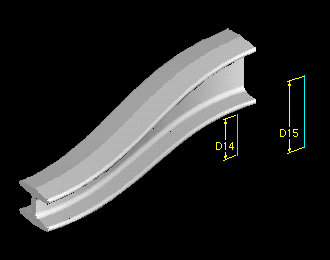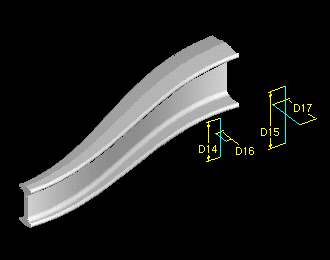>>>  Go to Pro/E Tips Index  >>>
>>>  Page to Previous Pro/E Tip  >>>

JUNE 1999

 Driving Equations from the Outside Equations used in relations are a very convenient way to build design intent into your models.  The equations can be used to drive relationships between features within a part and relationships between parts in an assembly. For our discussion this month we'll deal with part equations, and specifically the driving equations of a variable section sweep.  Figure 1 shows our example variable section sweep where the height of the "I" section is driven by the equation:   sd7=1.25-0.5*trajparFigure 1. As you can see, this is a simple, smooth way of changing the height of the "I" beam as it is swept along it's trajectory. The unfortunate part about using such an equation is that to modify the values of the height of the beam, (the 1.25 or the 0.5 in the equation) you must go to the proper RELATIONS menu and EDIT RELations.  If this is bothersome you could write a Pro/Program which requests the information with each regeneration ...   or ... USE THIS TRICK ! Just prior to the feature to be driven, insert a datum curve containing the dimensional values you wish to use as modifiable constants in the equation.  Figure 2 shows the datum curve inserted, with it's dimensions shown in symbolic form.  The corresponding equation controling the feature is:   sd7=D15-(D15-D14)*trajpar Where  d15=1.25  and  d14=0.75  -- the "I" heights at the beam ends. Notice that the symbolic values of the datum curve dimensions have been substituted for the constants in the previous equation.  In this way, the datum curve can be easily changed using MODIFY, thereby changing the sweep without editing the relation.Figure 2. This is an easy way to control values used in relations.  Figure 3 shows this same "I" beam now driven in 2 directions -- width and height, with the corresponding datum curve drivers also shown.Figure 3. If you're interested, here are the equations:   sd7=D15-(D15-D14)*trajpar  sd8=D17-(D17-D16)*trajpar  Now if we could just figure out how to build a little more flexibility into life's more complicated relationships ....   Have a wonderful month.  We'll see you again in July!

.

SYNTHESIS ENGINEERING SERVICES INC. :  (719) 380-1122